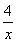A Quadratic Equation is one that can be written in the standard form ax2 + bx c = 0, where ab, and c are real numbers and a does not equal zero.

In any quadratic equation, the highest power of an unknown quantity is 2.
A quadratic equation can contain one or more second power variables.
A quadratic equation has at most two solutions.
The graph of a quadratic equation is a parabola.

### Video Examples: Introduction to the quadratic equation

7x2 + 9x + 2 = 0 is a quadratic equation, because this equation is in the form ax2 + bx + c = 0, where a = 7, b = 9, and c = 2 and the variable is a second degree variable.

### Solved Example on Quadratic Equation

#### Ques: Which of the following is a quadratic equation?

##### Choices:

A. x2 + 5x + 1 = 0
B. (3x - 1) (2x + 1) (x + 3) = 0
C. x += x2
D. x (x2 - 1) - 4x + 28 = 0